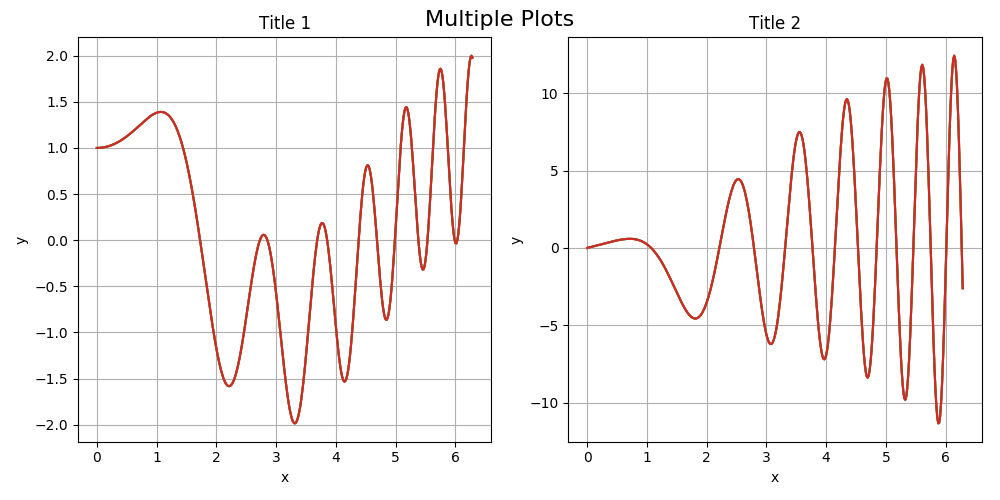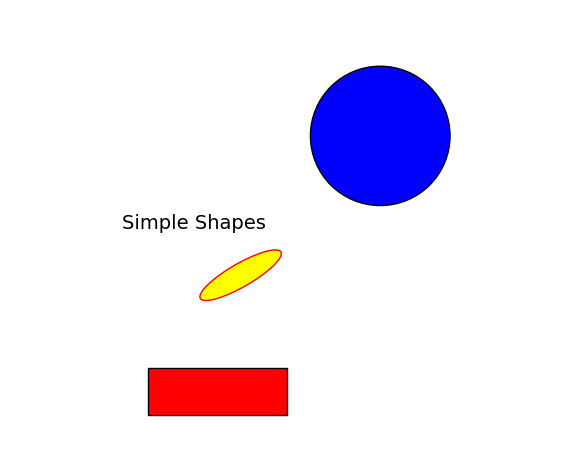# Tutorial 4 -- Graphical Outputs in Python

In this tutorial, we learn how to produce graphical outputs in python with the easy-to-use, yet sophisticated Matplotlib module. This review is limited to the pyplot submodule which can be imported with the following statement

import matplotlib.pyplot as plt


matplotlib.pyplot is a collection of plotting functions that make matplotlib work like MATLAB.

The documentation can be found here: Matplotlib User Guide. You will find many simple and complex illustrations of what this module can produce.

### Simple Plots from Arrays of Points

In this example, the pyplot submodule is used to produce a simple y vs x plot from data written in the form of two (1D) numpy arrays. We can easily add labels and a title, along with a grid. The default format string is ‘b-', which is a solid blue line. Three different plotting options are shown. There are many other available options.

import matplotlib.pyplot as plt
import numpy as np

x = np.arange(0.0, 4.0*np.pi, 0.1) # array of x values from 0 to 4*pi with increment of 0.1
y = 1 + np.sin(x)  # array of y values

plt.plot(x, y) # plot y vs x with continuous line (default)
plt.plot(x+np.pi/4, y, 'r.') # red (r) isolated points (.): the previous plot is shifted along x-axis by pi/4
plt.plot(x+np.pi/2, y, 'gx') # green (g) et cross (x)
plt.xlabel('x(m): distance traveled')
plt.ylabel('y(m): height')
plt.title('Oscillatory Motion of P')
plt.grid()
plt.savefig('x-y.png') # save graph in png file
plt.show()


The result is shown below (the default did not produce a solid blue line):You could also replace the 3 plot commands by a single one:

plt.plot(x, y, 'r--', x+np.pi/4, y, 'bs', x+np.pi/2, y, 'g^')


### Multiple Plots

You can plot multiple graphs with matplotlib. Here is a simple script where two graphs are plotted side by side.

import matplotlib.pyplot as plt
import numpy as np

#-- figure setup

fig = plt.figure(1, figsize=(10, 5)) # size in inches
plt.subplots_adjust(left=0.1, wspace=0.4, top= 0.8)
plt.suptitle('Multiple Plots', fontsize=16)

# plot figure 1
x = np.linspace(0, 2*np.pi, 400)
y1 = np.sin(x**2) + np.cos(x)
fig1 = plt.subplot(121) # nbr of rows =1, nbr of columns =2, plot_number =1
plt.title('Title 1')
plt.xlabel('x')
plt.ylabel('y')
plt.grid(True)
fig1.plot(x, y1)

# plot figure 2
y2 = 2 * x * np.cos(x**2) - np.sin(x)
fig2 = plt.subplot(122) # nbr of rows =1, nbr columns =2, plot_number =2
plt.title('Title 2')
plt.xlabel('x')
plt.ylabel('y')
plt.grid(True)
fig2.plot(x,y2)

plt.tight_layout() # this makes sure that the figures are not overlaid
plt.savefig('x-y2.png') # save graph in png file### Plot Simple Geometric Objects

Matplotlib allows you to create simple geometric objects (which could later be animated!). Here is a simple script with text, circle, ellipse and rectangle objects.

import matplotlib.pyplot as plt
import matplotlib.patches as mpatches

fig, ax = plt.subplots()

# plot some text
x,y = 0.1, 0.4
plt.text(x, y, 'Simple Shapes', ha="center", family='sans-serif', size=14)

# plot a circle
x,y = 0.5,0.6
r = 0.15
circ = plt.Circle((x,y), r, facecolor= 'blue', edgecolor= 'black')

# plot an ellipse
x,y = 0.2,0.3
rx,ry = 0.2, 0.05
angle = 30 # (degrees)
elli = mpatches.Ellipse((x,y), rx, ry, angle, facecolor= 'yellow', edgecolor= 'red')